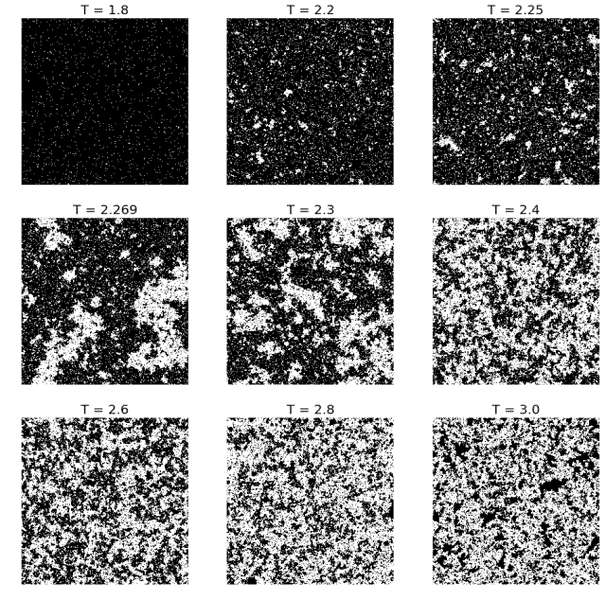# Convergence of lattice Ising model

Hello everyone.
I'm working on a program to solve 2D Ising model of magnetic materials, using a system with 10x10 spins for simplicity at a temperature of 1E-8 K. I'm using this parameters to get a faster result of m=1 and guarantee it is correct. but.......

For now i already pass 300 Monte Carlo's moves and it is in the same position of when it was in 50... is that normal ??? I've watch a vídeo on youtube where they took like a 10 million moves to compute a 200x200 system. I'm a bit scared with the time it will cost me.

Thanks.

Could I check what your initial state was? If you started with an arbitrary random state and immediately put it at such a low temperature, it is highly likely for the system to become stuck in a local equilibrium. Most of the Monte Carlo moves will be rejected because the extremely low temperature essentially prevents moves that increase the energy of the system from occurring.

Also, it is also worth noting that such Monte Carlo simulations are often measured in terms of lattice sweeps (total number of moves / number of lattice sites) - the idea being that in each update one should allow for every lattice spin to have a chance to flip on average.

Could I check what your initial state was? If you started with an arbitrary random state and immediately put it at such a low temperature, it is highly likely for the system to become stuck in a local equilibrium. Most of the Monte Carlo moves will be rejected because the extremely low temperature essentially prevents moves that increase the energy of the system from occurring.

Also, it is also worth noting that such Monte Carlo simulations are often measured in terms of lattice sweeps (total number of moves / number of lattice sites) - the idea being that in each update one should allow for every lattice spin to have a chance to flip on average.

yes, i've made it totally random and in it first move it was already at such temperature.. So, how should i proceed ? Low the temperature gradually ?

I usually prefer the alternative - to heat the system up instead. So instead of starting from a random state, I start with the zero temperature limit of perfectly aligned spins. This avoids the problem of getting trapped in local minima.

I usually prefer the alternative - to heat the system up instead. So instead of starting from a random state, I start with the zero temperature limit of perfectly aligned spins. This avoids the problem of getting trapped in local minima.

Not sure if i've got it. So, you start your system at a temperature of approximate 0 ( not exactly zero because of the exponential of delta E / T ), then you heat the system untill what temperature ? It cannot pass 2.6..something Kelvin because i want to observe the consequence of phase transition.

Not sure what it is that you want to achieve but here is a set of pictures of representative states of a Ising system at different temperatures that I've done in the past:You can either start with the uniformly aligned state each time or use the final output system state of the lower temperature as the starting state for the next (higher temperature) simulation.

However, it must be noted that these are just representative states of the system. In order to "observe" the phase transition, one has to measure other quantities such as the average magnetization and the susceptibility, which are averaged over the Monte Carlo runs.